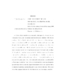## Orbit discontinuities and topological models for Borel semiflows##### Files
umi-umd-3231.pdf(452.47 KB)
Let $T_t$ be a Borel semiflow on a standard Polish space $X$. We say two distinct points $x$ and $y$ are instantaneously discontinuously identified'' (IDI) by the semiflow if $T_t(x) = T_t(y)$ for all $t &gt; 0$. We define the concept of orbit discontinuity'', a generalization of IDI, and examine the prevalence and structure of orbit discontinuities for arbitrary Borel semiflows. In particular we show that points have only countably many orbit discontinuities and that the set of orbit discontinuities has measure zero with respect to any measure preserved by the semiflow. Additionally, if the semiflow preserves a Borel probability measure on $X$, we show that the Ambrose-Kakutani theorem can be adapted to find both an extension and a factor of the semiflow which are conjugate to the original semiflow except on a set of measure zero. Both the factor and extension are characterized by a Polish space called the base'' with a vertical semiflow consisting of repeated quotient maps onto successively larger closed subsets of the base together with a return-time transformation describing how points return to the base. The points where the conjugacy fails are the orbit discontinuities of the original semiflow. Furthermore, we develop the concept of orbit discontinuity'' from a measure-theoretic perspective. Assuming $T_t$ preserves a Borel probability measure $\mu$ on $X$, we show that for all points $x$ in an invariant set of full $\mu-$measure, there exist two &quot;measure paths&quot; $\mu_{x,t}^+$ and $\mu_{x,t}^-$ which give, for almost every time $t$, a natural distribution on the set of points $y$ with $T_t(x) = T_t(y)$. These measures are constructed by taking weak$^*-$ limits of conditional expectations. We show that these measure paths coincide and are weak$^*-$ continuous except at countably many times $t$. If the measure paths differ at $t = 0$ for some point $x$, then $x$ has an orbit discontinuity at time $0$.